# Chapter 17: D.c. transients

### Example 1, page no. 262

In :
from __future__ import division
import math
#from pylab import *
%matplotlib inline
#initializing  the  variables:
C  =  15E-6;#  in  Farads
R  =  47000;#  in  ohms
V  =  120;#  in  Volts

#calculation:
tou  =  R*C
t1  =  tou
Vctou  =  V*(1-math.e**(-1*t1/tou))
Vct  =  V/2
t0  =  -1*tou*math.log(1  -  Vct/V)
t=[]
Vc=[]
I  =  V/R
for h in range(50):
t.append((h-1)/10)
k=(h-1)/10
Vc.append(V*(1 - math.e**(-1*k/tou)))
fig  = plt.figure()
ax = fig.add_subplot(1, 1, 1)
ax.plot(t,Vc,'-')
#plot(t,Vc,'-')
xlabel('time(sec)')
ylabel('Volts(V)')
show()

#Results
print  "\n\n  Result  \n\n"
print  "\n  (a)the  capacitor  voltage  at  a  time  equal  to  one  time  constant  =  ",round(Vctou,2),"  V"
print  "\n  (b)the  time  for  the  capacitor  voltage  to  reach  one  half  of  its  steady  state  value  =  ",round(t0,5)," secs"

Populating the interactive namespace from numpy and matplotlib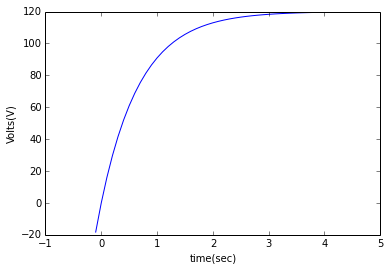Result

(a)the  capacitor  voltage  at  a  time  equal  to  one  time  constant  =   75.85   V

(b)the  time  for  the  capacitor  voltage  to  reach  one  half  of  its  steady  state  value  =   0.48867  secs


### Example 2, page no. 263

In :
from __future__ import division
import math
#from pylab import *
%matplotlib inline
#initializing  the  variables:
C  =  4E-6;#  in  Farads
R  =  220000;#  in  ohms
V  =  24;#  in  Volts
t1  =  1.5;#  in  secs

#calculation:
tou  =  R*C
I  =  V/R

t=[]
Vc=[]
for h in range(50):
t.append((h-1)/10)
k=(h-1)/10
Vc.append(V*math.e**(-1*k/tou))
#plt.figsize(10,8)
fig  = plt.figure()
#canvas = fc(fig)
ax = fig.add_subplot(1, 1, 1)
ax.plot(t,Vc,'-')
#plot(t,Vc,'-')
xlabel('time(sec)')
ylabel('P.D across Capacitor(V)')
show()

t=[]
VR=[]
for h in range(50):
t.append((h-1)/10)
k=(h-1)/10
VR.append(V*(1 - math.e**(-1*k/tou)))
fig  = plt.figure()
#canvas = fc(fig)
ax = fig.add_subplot(1, 1, 1)
ax.plot(t,VR,'-')
#plot(t,VR,'-*')
xlabel('time(sec)')
ylabel('P.D across Resistor(V)')
show()

t=[]
i=[]
for h in range(50):
t.append((h-1)/10)
k=(h-1)/10
i.append(I*math.e**(-1*k/tou))
fig  = plt.figure()
#canvas = fc(fig)
ax = fig.add_subplot(1, 1, 1)
ax.plot(t,i,'-')
#plot(t,i,'*-')
xlabel('time(sec)')
ylabel('current(A)')
show()

Vct1  =  V*math.e**(-1*t1/tou)
VRt1  =  V*math.e**(-1*t1/tou)
it1   =  I*math.e**(-1*t1/tou)

#Results
print  "\n\n  Result  \n\n"
print  "\n  the  value  of  capacitor  voltage  is  ",round(Vct1,1),"  V,  resistor  voltage  is  ",round(VRt1,1),"  V,"
print  "current  is  ",round(0.02,2),"  mA  at  one  and  a  half  seconds  after  discharge  has  started."

Populating the interactive namespace from numpy and matplotlib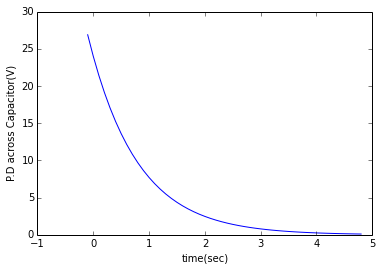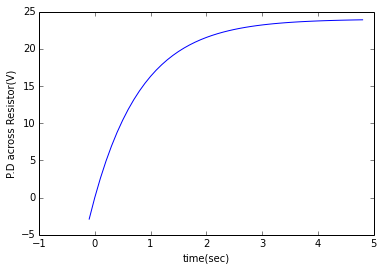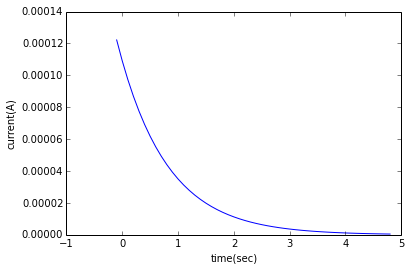Result

the  value  of  capacitor  voltage  is   4.4   V,  resistor  voltage  is   4.4   V,
current  is   0.02   mA  at  one  and  a  half  seconds  after  discharge  has  started.


### Example 3, page no. 265

In :
from __future__ import division
import math
#initializing  the  variables:
C  =  20E-6;#  in  Farads
R  =  50000;#  in  ohms
V  =  20;#  in  Volts
t1  =  1;#  in  secs
t2  =  2;#  in  secs
VRt  =  15;#  in  Volts

#calculation:
tou  =  R*C
I  =  V/R
Vct1  =  V*(1-math.e**(-1*t2/tou))
t3  =  -1*tou*math.log(VRt/V)
it1  =  I*math.e**(-1*t1/tou)

#Results
print  "\n\n  Result  \n\n"
print  "\n  (a)initial  value  of  the  current  flowing  is  ",round(I*1000,1),"mA"
print  "\n  (b)time  constant  of  the  circuit  ",round(tou,2),"  Sec"
print  "\n  (c)the  value  of  the  current  one  second  after  connection,  ",round((it1/1E-3),3),"  mA"
print  "\n  (d)the  value  of  the  capacitor  voltage  two  seconds  after  connection  ",round(Vct1,1),"  V"
print  "\n  (e)the  time  after  connection  when  the  resistor  voltage  is  15  V  is  ",round(t3,3),"  sec"


Result

(a)initial  value  of  the  current  flowing  is   0.4 mA

(b)time  constant  of  the  circuit   1.0   Sec

(c)the  value  of  the  current  one  second  after  connection,   0.147   mA

(d)the  value  of  the  capacitor  voltage  two  seconds  after  connection   17.3   V

(e)the  time  after  connection  when  the  resistor  voltage  is  15  V  is   0.288   sec


### Example 17.4, page no. 266

In :
from __future__ import division
import math
#initializing  the  variables:
C  =  0.5E-6;#  in  Farads
V  =  10;#  in  Volts
tou  =  0.012;#  in  secs
t1  =  0.007;#  in  secs

#calculation:
R  =  tou/C
Vc  =  V*(1-math.e**(-1*t1/tou))

#Results
print  "\n\n  Result  \n\n"
print  "\n  (a)value  of  the  resistor  is  ",R,"  ohm"
print  "\n  (b)capacitor  voltage  is  ",round(Vc,2),"  V"


Result

(a)value  of  the  resistor  is   24000.0   ohm

(b)capacitor  voltage  is   4.42   V


### Example 5, page no. 267

In :
from __future__ import division
import math
#initializing  the  variables:
R  =  50000;#  in  ohms
V  =  100;#  in  Volts
Vc1  =  20;#  in  Volts
tou  =  0.8;#  in  secs
t1  =  0.5;#  in  secs
t2  =  1;#  in  secs

#calculation:
C  =  tou/R
t  =  -1*tou*math.log(Vc1/V)
I  =  V/R
it1  =  I*math.e**(-1*t1/tou)
Vc  =  V*math.e**(-1*t2/tou)

#Results
print  "\n\n  Result  \n\n"
print  "\n  (a)the  value  of  the  capacitor  is  ",round((C/1E-6),2),"uF"
print  "\n  (b)the  time  for  the  capacitor  voltage  to  fall  to  20  V  is  ",round(t,2),"  sec"
print  "\n  (c)the  current  flowing  when  the  capacitor  has  been  discharging  for  0.5  s  is  ",round(it1*1000,2),"mA"
print  "\n  (d)voltage  drop  across  resistor  when  the  capacitor  has  been  discharging  for  one  second  is  ",round(Vc,1),"  V"


Result

(a)the  value  of  the  capacitor  is   16.0 uF

(b)the  time  for  the  capacitor  voltage  to  fall  to  20  V  is   1.29   sec

(c)the  current  flowing  when  the  capacitor  has  been  discharging  for  0.5  s  is   1.07 mA

(d)voltage  drop  across  resistor  when  the  capacitor  has  been  discharging  for  one  second  is   28.7   V


### Example 6 page no. 268

In :
from __future__ import division
import math
#initializing  the  variables:
C  =  0.1E-6;#  in  Farads
R  =  4000;#  in  ohms
V  =  200;#  in  Volts
Vc1  =  2;#  in  Volts

#calculation:
tou  =  R*C
I  =  V/R
t  =  -1*tou*math.log(Vc1/V)

#Results
print  " \n\n  Result  \n\n"
print  "\n  (a)  initial  discharge  current  is  ",round(I,2),"  A"
print  "\n  (b)Time  constant  tou  is  ",round(tou,5),"  sec"
print  "\n  (c)min.  time  required  for voltage  across capacitor  to  fall  to  less  than  2  V  is  ",round(t*1000,0),"  msec"



Result

(a)  initial  discharge  current  is   0.05   A

(b)Time  constant  tou  is   0.0004   sec

(c)min.  time  required  for voltage  across capacitor  to  fall  to  less  than  2  V  is   2.0   msec


### Example 7, page no. 270

In :
from __future__ import division
import math
from pylab import *
#initializing  the  variables:
L  =  0.1;#  in  Henry
R  =  20;#  in  ohms
V  =  60;#  in  Volts
i2  =  1.5;#  in  Amperes

#calculation:
tou  =  L/R
t1  =  2*tou

t=[]
i=[]
I  =  V/R
for h in range(250):
t.append((h-1)/10000)
k=(h-1)/10000
i.append(I*(1  -  math.e**(-1*k/tou)))
plot(t,i,'-')
xlabel('time(sec)')
ylabel('current(A)')
show()
i1  =  I*(1  -  math.e**(-1*t1/tou))
t2  =  -1*tou*math.log(1  -  i2/I)

#Results
print  " \n\n  Result  \n\n"
print  "\n  (a)  the  value  of  current  flowing  at  a  time  equal  to  two  time  constants  is  ",round(i1,2),"  A"
print  "\n  (b)the  time  for  the  current  to  grow  to  1.5  A  is  ",round(t2,5),"  sec"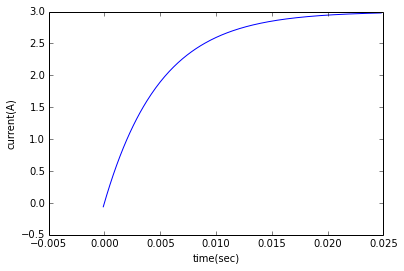Result

(a)  the  value  of  current  flowing  at  a  time  equal  to  two  time  constants  is   2.59   A

(b)the  time  for  the  current  to  grow  to  1.5  A  is   0.00347   sec


### Example 8, page no. 271

In :
from __future__ import division
import math
#initializing  the  variables:
L  =  0.04;#  in  Henry
R  =  10;#  in  ohms
V  =  120;#  in  Volts

#calculation:
tou  =  L/R
t1  =  tou
I  =  V/R
i1  =  I*(1  -  math.e**(-1*t1/tou))
i2  =  0.01*I
t2  =  -1*tou*(-5)

#Results
print  "\n\n  Result  \n\n"
print  "\n  (a)  the  final  value  of  current  is  ",round(I,2),"  A"
print  "\n  (b)time  constant  of  the  circuit  is  ",round(tou*1000,2),"msec"
print  "\n  (c)  value  of  current  after  a  time  equal  to  the  time  constant  is  ",round(i1,2),"  A"
print  "\n  (d)expected  time  for  current  to  rise  to  within  0.01  times  of  its  final  value  is  ",round(t2*1000,2),"msec"


Result

(a)  the  final  value  of  current  is   12.0   A

(b)time  constant  of  the  circuit  is   4.0 msec

(c)  value  of  current  after  a  time  equal  to  the  time  constant  is   7.59   A

(d)expected  time  for  current  to  rise  to  within  0.01  times  of  its  final  value  is   20.0 msec


### Example 9, page no. 271

In :
from __future__ import division
import math
#initializing  the  variables:
L  =  3;#  in  Henry
R  =  15;#  in  ohms
V  =  120;#  in  Volts
t1  =  0.1;#  in  secs
t3  =  0.3;#  in  secs

#calculation:
tou  =  L/R
I  =  V/R
i2  =  0.85*I;#  in  amperes
VL  =  V*math.e**(-1*t1/tou)
t2  =  -1*tou*math.log(1  -  (i2/I))
i3  =  I*(1  -  math.e**(-1*t3/tou))

#Results
print  "\n\n  Results  \n\n"
print  "\n  (a)  steady  state  value  of  current  is  ",round(I,2),"  A"
print  "\n  (b)time  constant  of  the  circuit  is  ",round(tou,2),"  sec"
print  "\n  (c)value  of  the  induced  e.m.f.  after  0.1  s  is  ",round(VL,2),"  V"
print  "\n  (d)  time  for  the  current  to  rise  to  0.85  times  of  its  final  values  is  ",round(t2,2),"  A"
print  "\n  (e)value  of  the  current  after  0.3  s  is  ",round(i3,2),"  A"


Results

(a)  steady  state  value  of  current  is   8.0   A

(b)time  constant  of  the  circuit  is   0.2   sec

(c)value  of  the  induced  e.m.f.  after  0.1  s  is   72.78   V

(d)  time  for  the  current  to  rise  to  0.85  times  of  its  final  values  is   0.38   A

(e)value  of  the  current  after  0.3  s  is   6.21   A


### Example 10, page no. 273

In :
from __future__ import division
import math
from pylab import *
#initializing  the  variables:
R  =  15;#  in  ohms
V  =  110;#  in  Volts
tou  =  2;#  in  secs
t1  = 3;#  in  secs
i2  = 5;#  in  amperes

#calculation:
L  =  tou*R
I  =  V/R

t=[]
i=[]
for h in range(100):
t.append((h-1)/10)
k=(h-1)/10
i.append(I*math.e**(-1*k/tou))
plot(t,i,'-')
xlabel('time(sec)')
ylabel('current(A)')
show()
i1  =  I*(math.e**(-1*t1/tou))
t2  =  -1*tou*log((i2/I))

#Results
print  " \n\n  Result  \n\n"
print  "\n  (a)the  current  flowing  in  the  winding  3  s  after  being  shorted-out  is  ",round(i1,2),"  A"
print  "\n  (b)the  time  for  the  current  to  decay  to  5  A  is  ",round(t2,2),"  sec"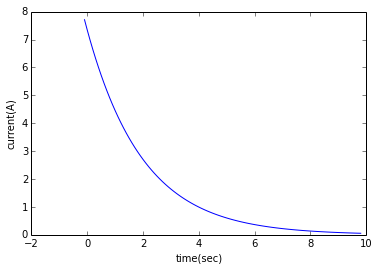Result

(a)the  current  flowing  in  the  winding  3  s  after  being  shorted-out  is   1.64   A

(b)the  time  for  the  current  to  decay  to  5  A  is   0.77   sec


### Example 11, page no. 273

In :
from __future__ import division
import math
#initializing  the  variables:
L  =  6;#  in  Henry
r  =  10;#  in  ohms
V  =  120;#  in  Volts
tou  =  0.3;#  in  secs
t1  =  1;#  in  secs

#calculation:
R  =  (L/tou)  -  r
Rt  =  R  +  r
I  =  V/Rt
i2  =  0.1*I
i1  =  I*(math.e**(-1*t1/tou))
t2  =  -1*tou*math.log((i2/I))

#Results
print  "  \n\n  Result  \n\n"
print  "\n  (a)  resistance  of  the  coil  is  ",round(R,2),"  ohm"
print  "\n  (b)  current  flowing  in  circuit  one  second  after  the  shorting  link  has  been  placed  is  ",round(i1,2),"  A"
print  "\n  (c)the  time  for  the  current  to  decay  to  0.1  times  of  initial  value  is  ",round(t2,2),"  sec"



Result

(a)  resistance  of  the  coil  is   10.0   ohm

(b)  current  flowing  in  circuit  one  second  after  the  shorting  link  has  been  placed  is   0.21   A

(c)the  time  for  the  current  to  decay  to  0.1  times  of  initial  value  is   0.69   sec


### Example 12, page no. 274

In :
from __future__ import division
import math
#initializing  the  variables:
L  =  0.2;#  in  Henry
R  =  1000;#  in  ohms
V  =  24;#  in  Volts
t1  =  1*L/R;#  in  secs
t2  =  2*L/R;#  in  secs
t3  =  3*L/R;#  in  secs

#calculation:
tou  =  L/R
I  =  V/R
i1  =  I*(1  -  math.e**(-1*t1/tou))
VL  =  V*(math.e**(-1*t2/tou))
VR  =  V*(1  -  math.e**(-1*t3/tou))

#Results
print  "\n\n  Result  \n\n"
print  "\n  time  constant  of  circuit  is  ",round(tou*1000,6),"msec, and steady-state  value  of current  is  ",round(I*1000,2),"mA"
print  "\n  (a)  urrent  flowing  in  the  circuit  at  a  time  equal  to  one  time  constant  is  ",round(i1*1000,2),"mA"
print  "\n  (b)  voltage  drop  across  the  inductor  at  a  time  equal  to  two  time  constants  is  ",round(VL,3),"  V"
print  "\n  (c)the  voltage  drop  across  the  resistor  after  a  time  equal  to  three  time  constants  is  ",round(VR,2),"  V"


Result

time  constant  of  circuit  is   0.2 msec, and steady-state  value  of current  is   24.0 mA

(a)  urrent  flowing  in  the  circuit  at  a  time  equal  to  one  time  constant  is   15.17 mA

(b)  voltage  drop  across  the  inductor  at  a  time  equal  to  two  time  constants  is   3.248   V

(c)the  voltage  drop  across  the  resistor  after  a  time  equal  to  three  time  constants  is   22.81   V